# Question & Answer: Data Table 1. Pressure and Temperature Measurements. Trial 1 Trial 2 Trial 3 Trial 4…..

I need help on making this graph. I do not understand how to do it at all. PLEASE!!!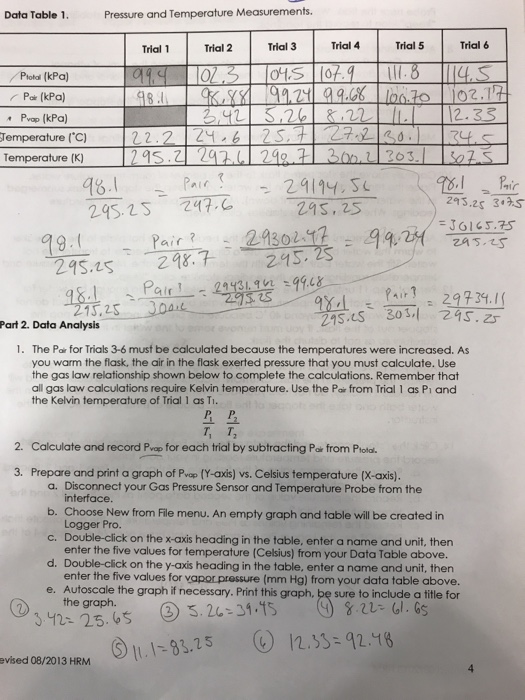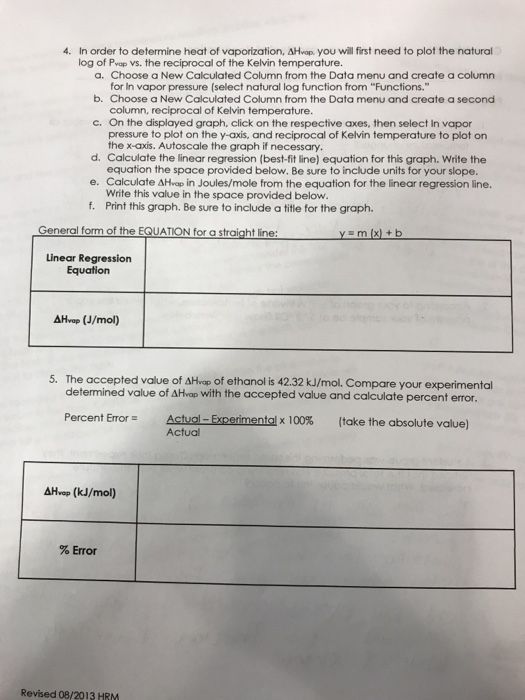Data Table 1. Pressure and Temperature Measurements. Trial 1 Trial 2 Trial 3 Trial 4 Trial 5 Trial 6 Piotal (kPa P (kPa) Pvap (kPa) Temperature (℃) as.z 263 29S.2S 24 24S,25 243.2s 34S 245.is 제7– 245.75 Pir 29734.1 21s.cS 301,1 275.25 13,25 Part 2. Data Analysis 1. The Pairfor Trials 3-6 must be calculated because the temperatures were increased. As you warm the flask, the air in the flask exerted pressure that you must calculate. Use the gas law relationship shown below to complete the calculations. Remember that all gas law calculations require Kelvin temperature. Use the Pair from Trial 1 as Pi and the Kelvin temperature of Trial 1 as T1. T. T 2. Calculate and record Pigp for each trial by subtracting Pai from Prod 3. Prepare and print a graph of Pvop (Y-axis) vs. Celsius temperature (X-axis). a. Disconnect your Gas Pressure Sensor and Temperature Probe from the b. Choose New from File menu. An empty graph and table will be created in c. Double-click on the x-axis heading in the table, enter a name and unit, then d. Double-click on the y-axis heading in the table, enter a name and unit, thern e. Autoscale the graph if necessary. Print this graph, be sure to include a title for interface Logger Pro. enter the five values for temperature (Celsius) from your Data Table above. enter the five values for vapor pressure (mm Hg) from your data table above. the graph. 312 25.6 3.26 ⑤1.1-83.25 (e) / 2.15-92.Y8 evised 08/2013 HRM

4. The graph in problem 4 states that we have to plot the log Pvap vs. the reciprocal of the Kelvin temperature, so that’s the only information we will need for this graph.

Don't use plagiarized sources. Get Your Custom Essay on
Question & Answer: Data Table 1. Pressure and Temperature Measurements. Trial 1 Trial 2 Trial 3 Trial 4…..
GET AN ESSAY WRITTEN FOR YOU FROM AS LOW AS \$13/PAGE

Start with a table like this, gathering all the data points from the information we need: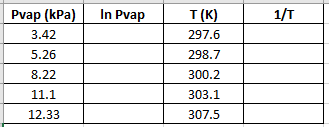Now, we need to calculate the lnPvap and the reciprocal of the temperature (1/T):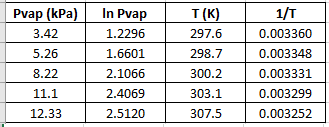Now, plot the graph with lnPvap as y and 1/T as x using a software of your choice (I’m using Excel):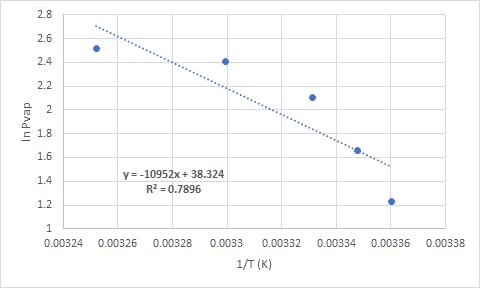Linear regression equation: -10952x + 38.324

To calculate ΔHvap we need only the value of m (-10952K) and we’ll use the formula:

m = -ΔHvap/R

Where R = 8.314 J/molK.

Solve for ΔHvap:

ΔHvap = -mR

ΔHvap = -(-10952K)(8.314J/molK)

ΔHvap = 91.05 kJ/mol

5. To calculate the error use the actual ΔHvap = 42.32 kJ/mol and the experimental value we obtained above and the given formula:

%error = ((91.05 kJ/mol – 42.32 kJ/mol) / 42.32 kJ/mol ) x 100

% error = 115%